#Function Repository Resource:

# RandomMandala

Make random mandala plots

Contributed by: Anton Antonov  |  Anton Antonov
 ResourceFunction["RandomMandala"][] generates a mandala graphic.

## Details and Options

The mandalas made by ResourceFunction["RandomMandala"] are generated through rotational symmetry of a "seed segment."
ResourceFunction["RandomMandala"] accepts the same options as Graphics as well as the following options to specify different features of the seed segment generation and rotational symmetry order:
 "RotationalSymmetryOrder" 6 a value k defining the rotation symmetry angle 2π/k "Radius" 10 maximum radius of the seed segment generation points "SymmetricSeed" True whether the seed should be symmetric "NumberOfSeedElements" Automatic the number of elements in a seed segment "KeepGridPoints" False should the grid points be shown "ConnectingFunction" Random how to connect a grid point "SeedFunction" Automatic a function for drawing the seed segment "ColorFunction" None how to colorize the mandala "FaceForm" {} face graphics directive for filled objects "EdgeForm" {} edge graphics directive for filled objects
The option "ConnectionFunction" specifies what Graphics primitive function should be used to connect the grid points of the seed segment. If the value is Automatic then Polygon is used. If the value is Random then a random choice is made from the following: Line, Polygon, BezierCurve or FilledCurve[BezierCurve[#]]&.
The option "SeedFunction" specifies the function for drawing mandala's seed segment. If the value is Automatic, then the seed segment is drawn by taking into account the values of the options "Radius", "NumberOfSeedElements", "KeepGridPoints" and "ConnectingFunction".

## Examples

### Basic Examples (2)

Here we generate a random mandala:

 In:=Out=Here we generate a mandala with different option settings:

 In:=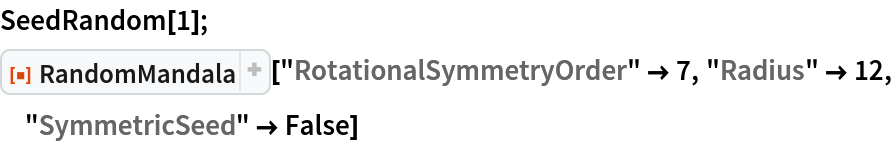Out=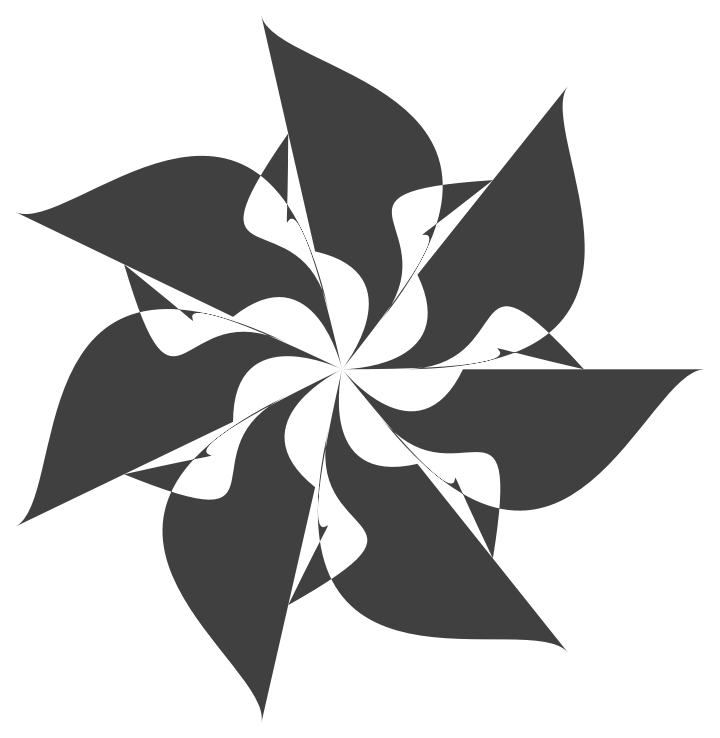### Scope (2)

There are two modes of making random mandalas: (i) single-mandala mode and (ii) multi-mandala mode. The multi-mandala mode is activated by giving the "Radius" option a list of positive reals.

Here are gray mandalas generated with the single-mandala mode:

 In:=Out=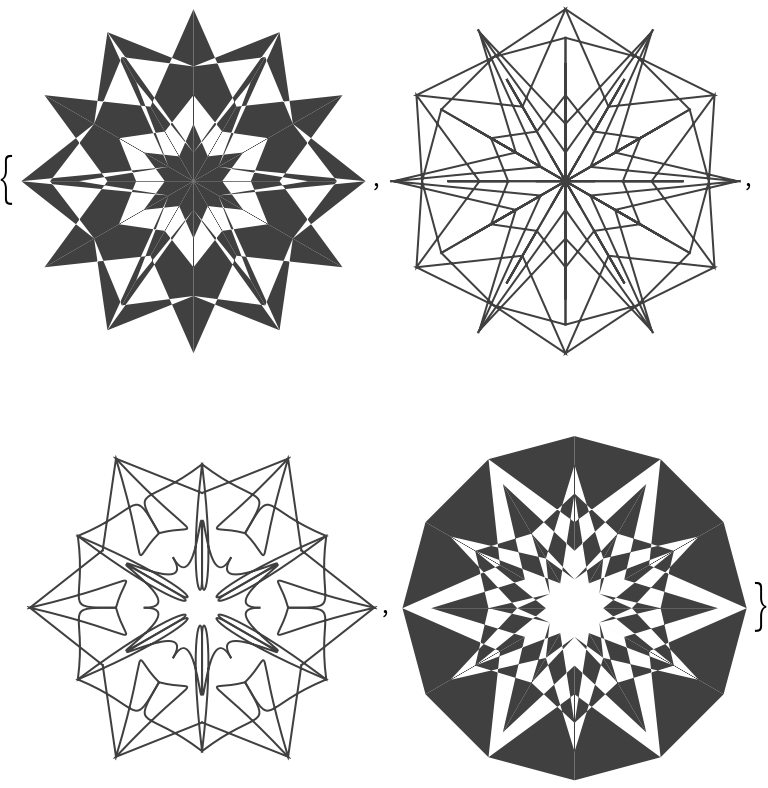Here are colorized mandalas generated with the single-mandala mode:

 In:=Out=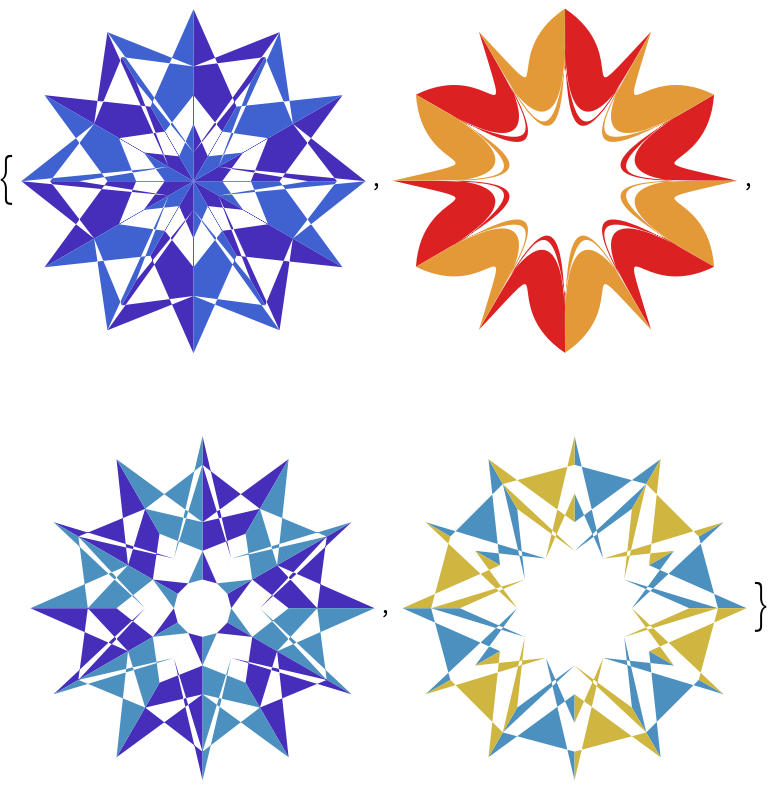Here are gray mandalas generated with a multi-mandala mode:

 In:=Out=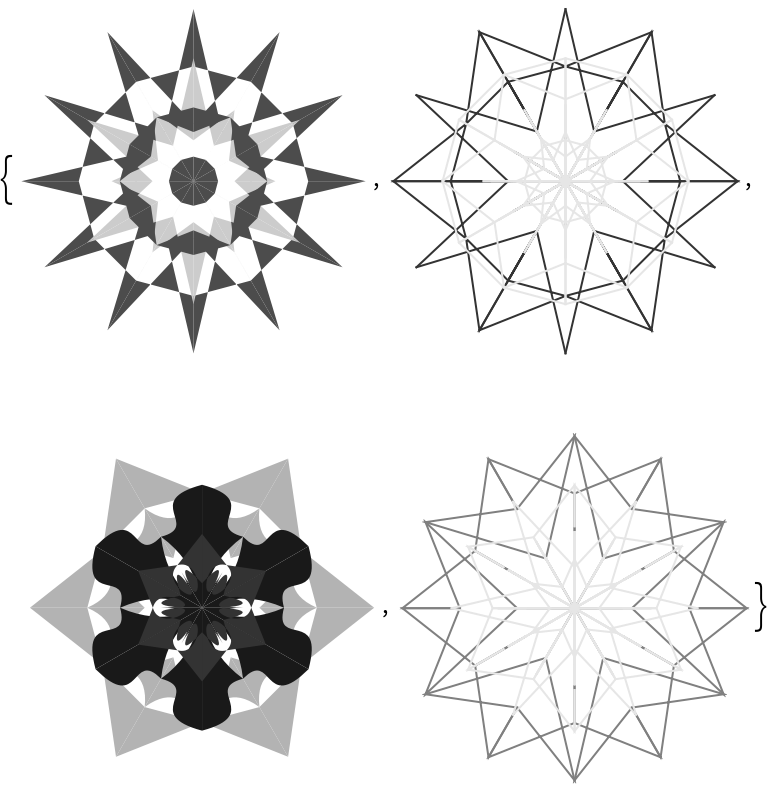Here are colorized mandalas generated with a multi-mandala mode:

 In:=Out=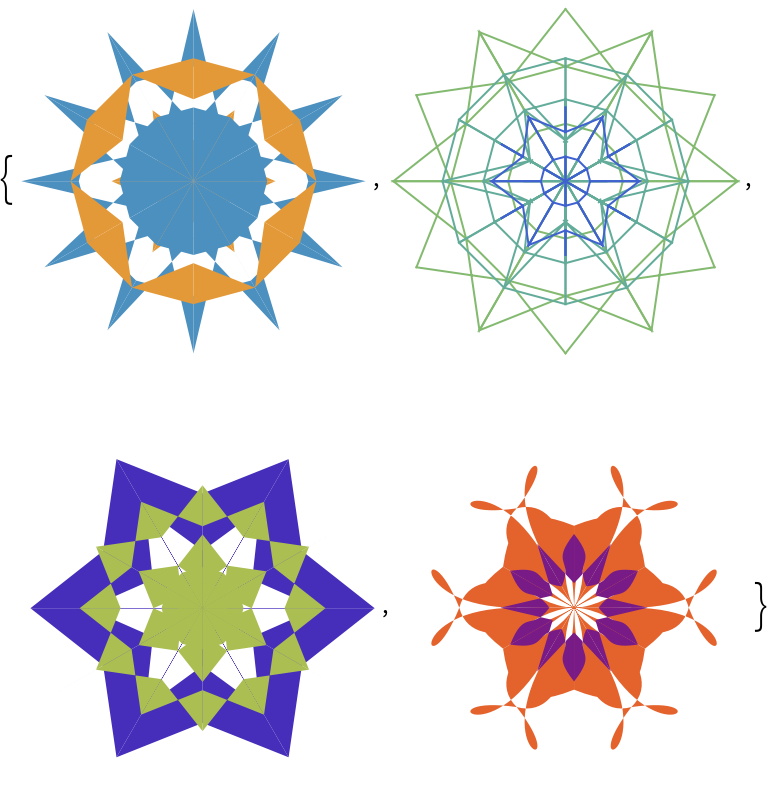### Options (15)

#### ColorFunction (2)

The function given to ColorFunction is run over Range[0,1,0.1] and the obtained colors are used to colorize the mandala. By default no colorizing is done and .

ColorFunction can also take strings that are color schemes known by ColorData.

Here is a list of colorized mandalas using single-mandala mode:

 In:=Out=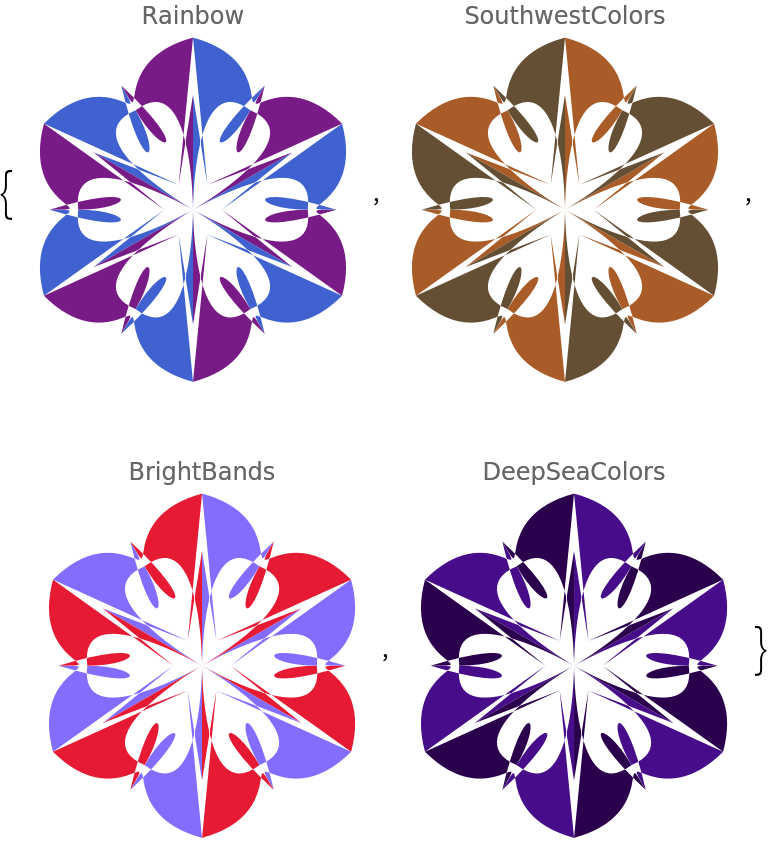Here are mandalas generated using multi-mandala mode:

 In:=Out=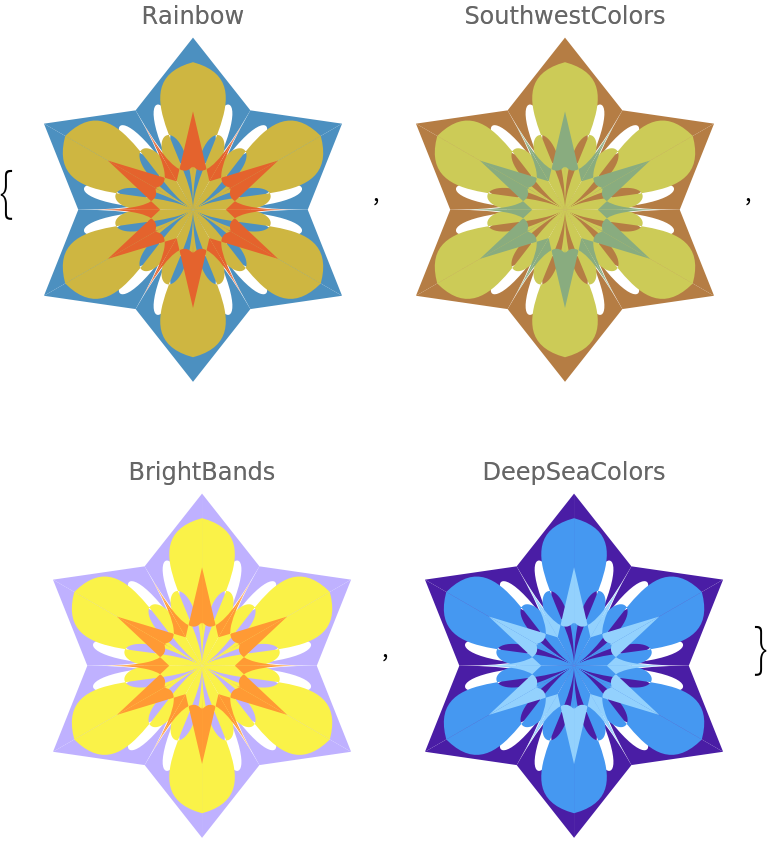#### ConnectingFunction (1)

The option "ConnectingFunction" specifies which graphics primitive to use over the seed segment points:

 In:=Out=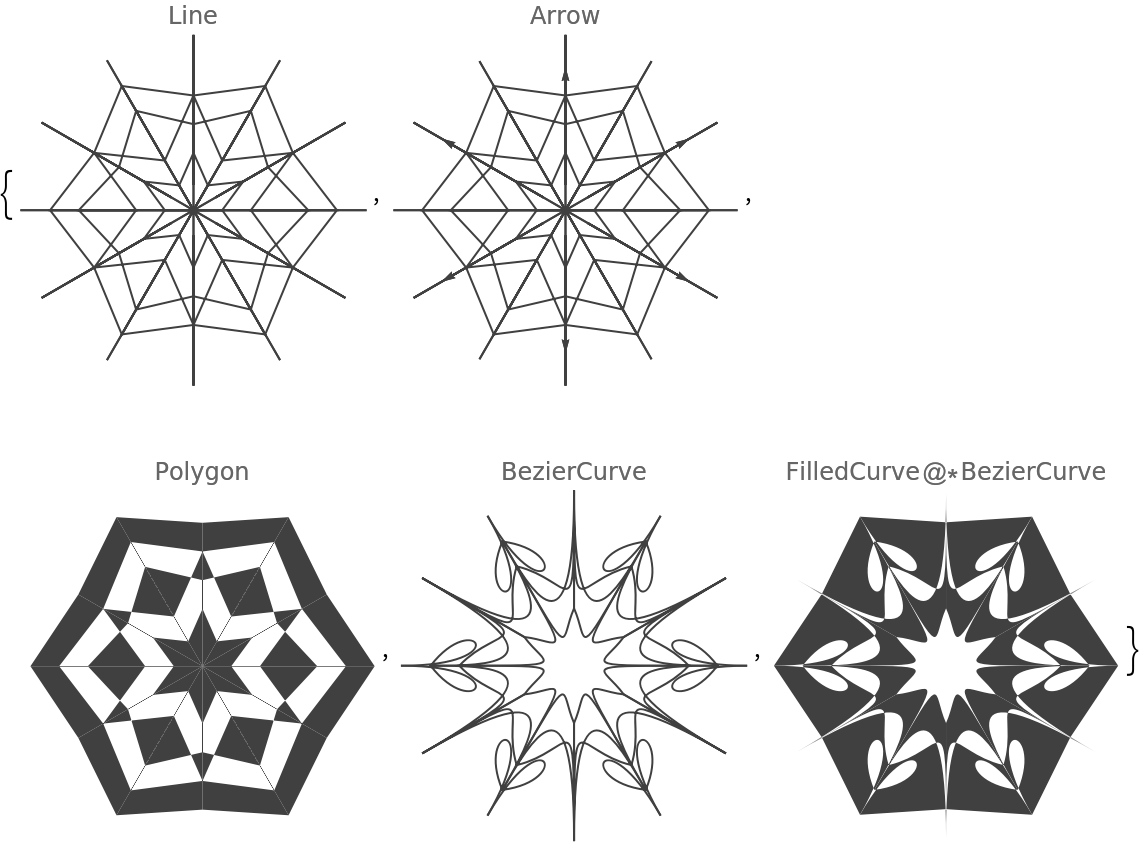#### EdgeForm (1)

The option EdgeForm specifies directives for the edges of polygons or other filled graphics objects:

 In:=Out=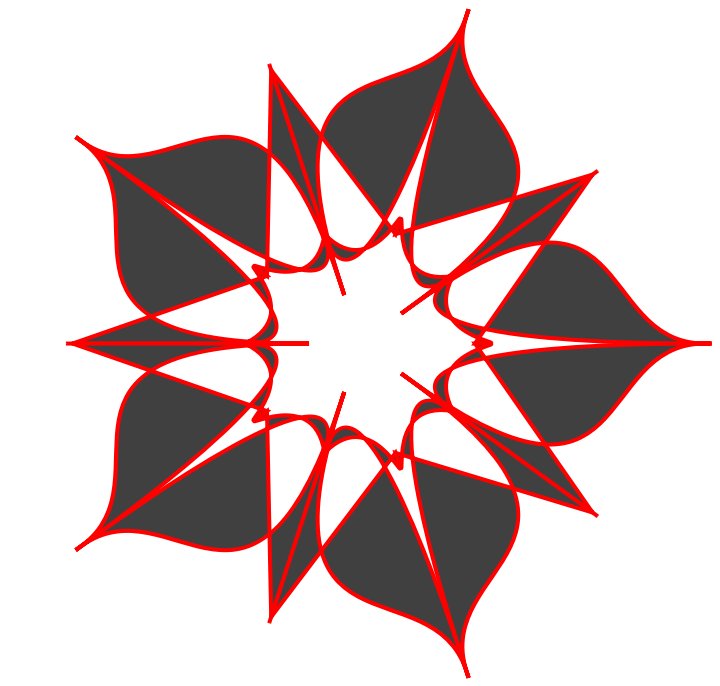#### FaceForm (1)

The option FaceForm specifies directives for the faces of polygons or other filled graphics objects:

 In:=Out=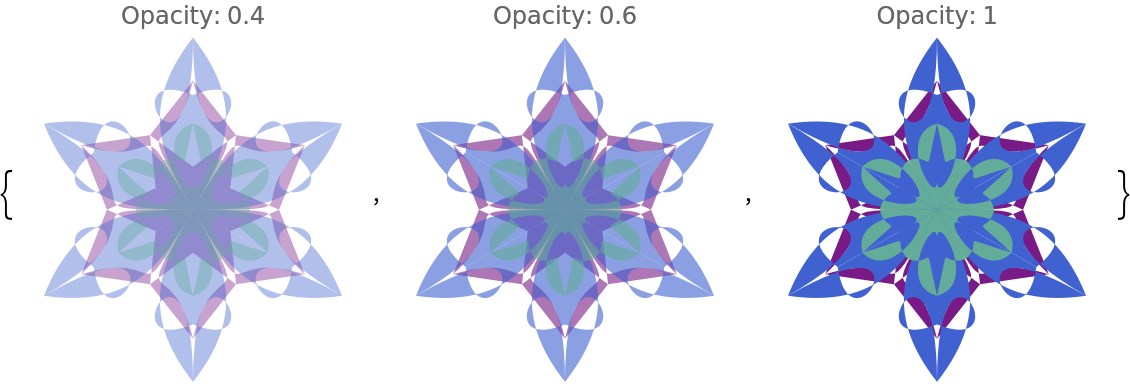#### KeepGridPoints (1)

The option "KeepGridPoints" specifies whether the points used to generate the seed segment should be kept:

 In:=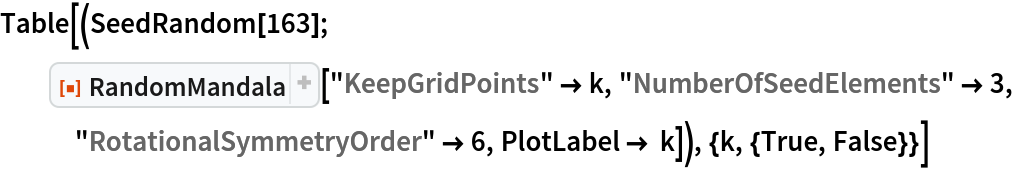Out=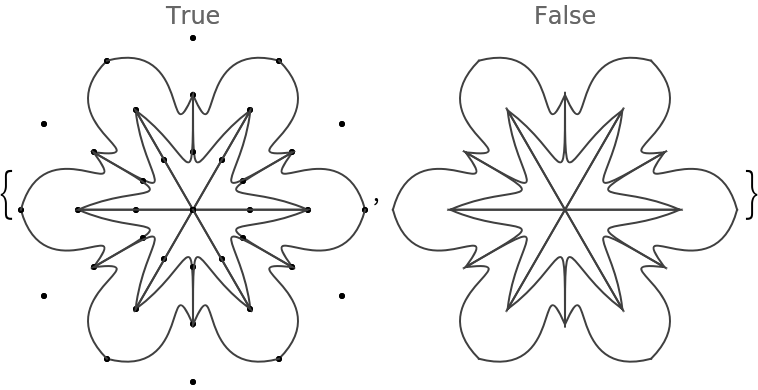In:=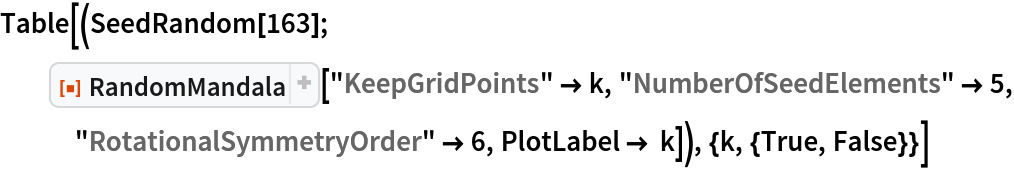Out=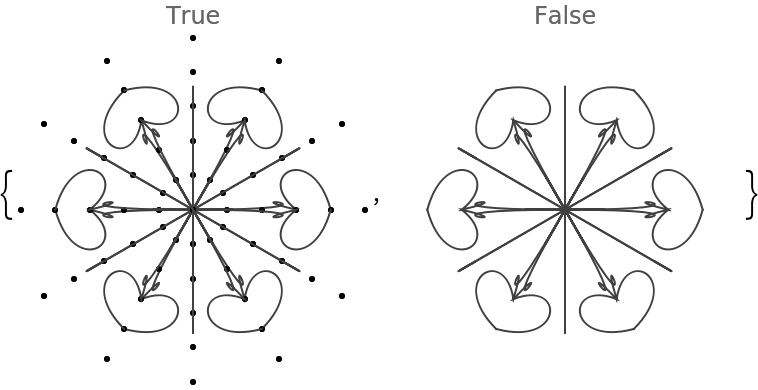#### NumberOfElements (1)

The option "NumberOfElements" controls how may graphics elements are in the seed segment:

 In:=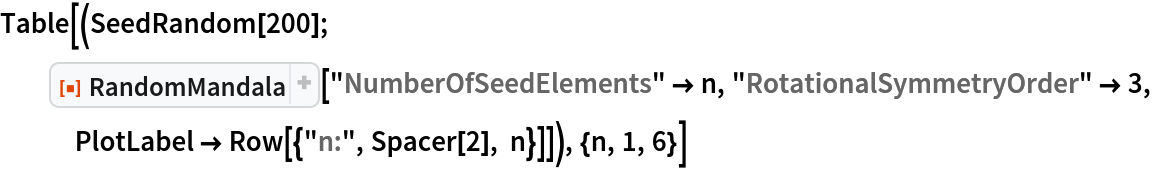Out=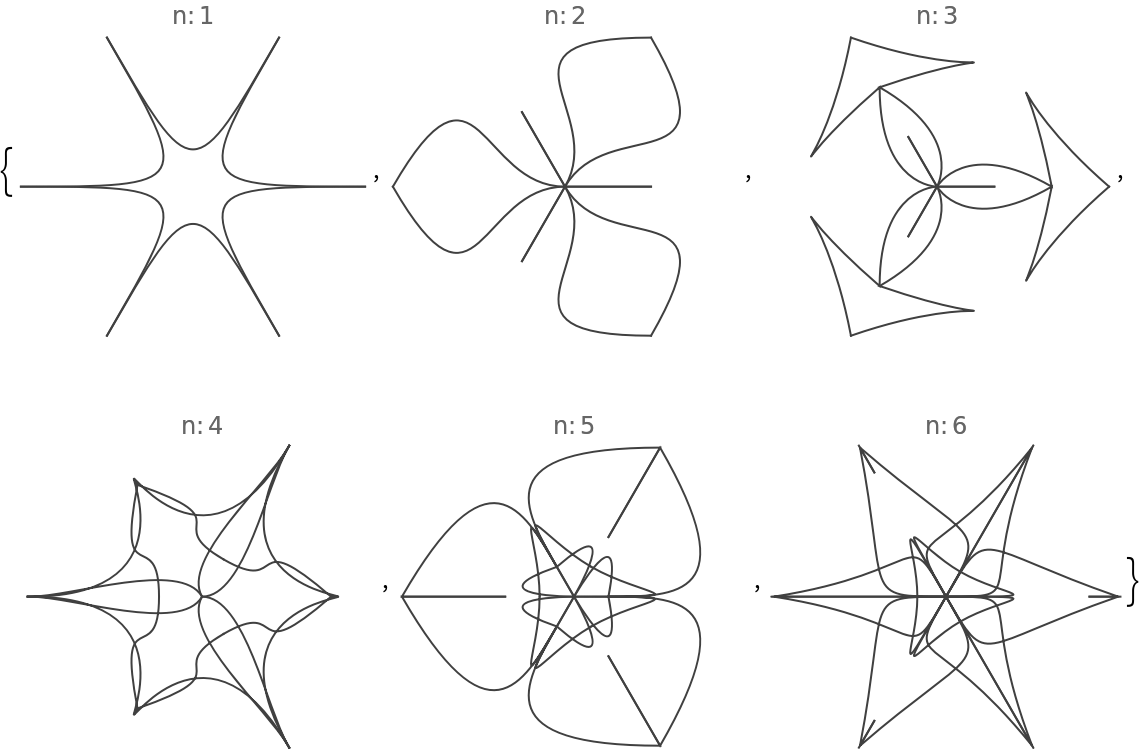In:=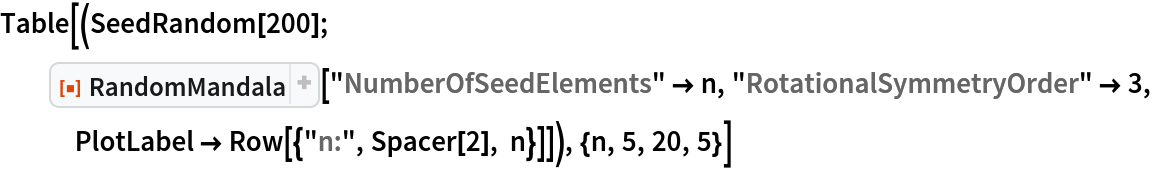Out=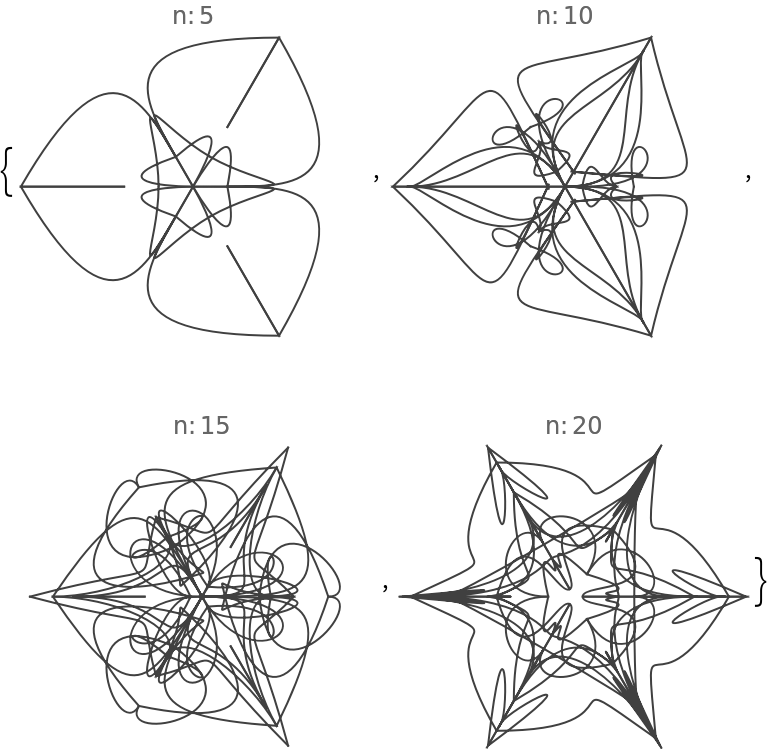If the value of "NumberOfElements" is Automatic in single-mandala mode, 6 elements are used; in multi-mandala mode, 3 elements are used (for each mandala).

In single-mandala mode the option "Radius" specifies the radius of the seed segment and the mandala:

 In:=Out=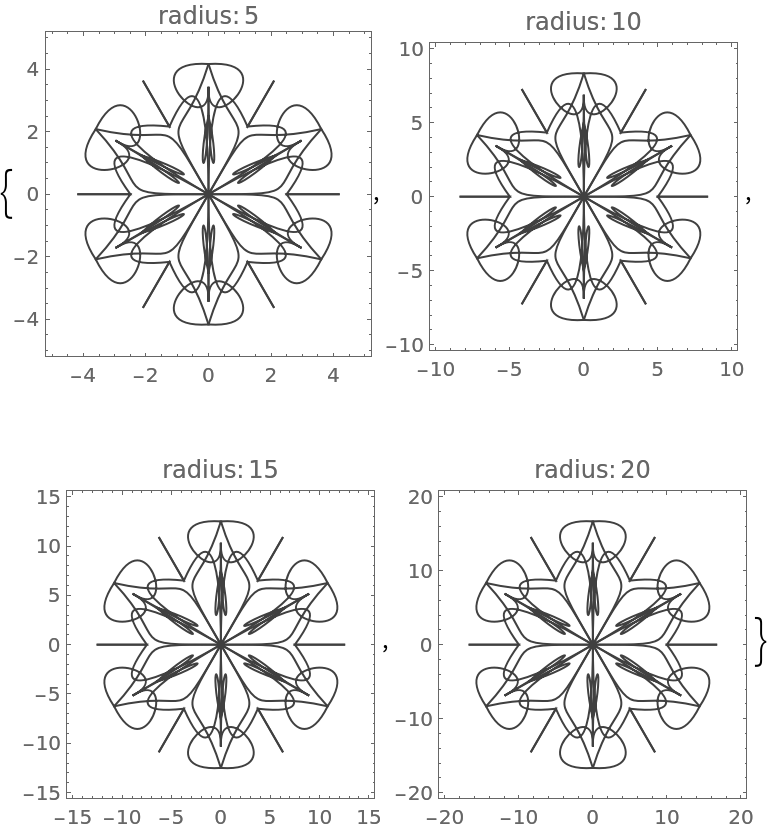If the value given to "Radius" is a list of positive numbers, then multi-mandala mode is used.

If "Radius"{r1,…,rk}, then for each ri, a mandala is made with radius ri and the mandalas are drawn upon each other according to their radii order:

 In:=Out=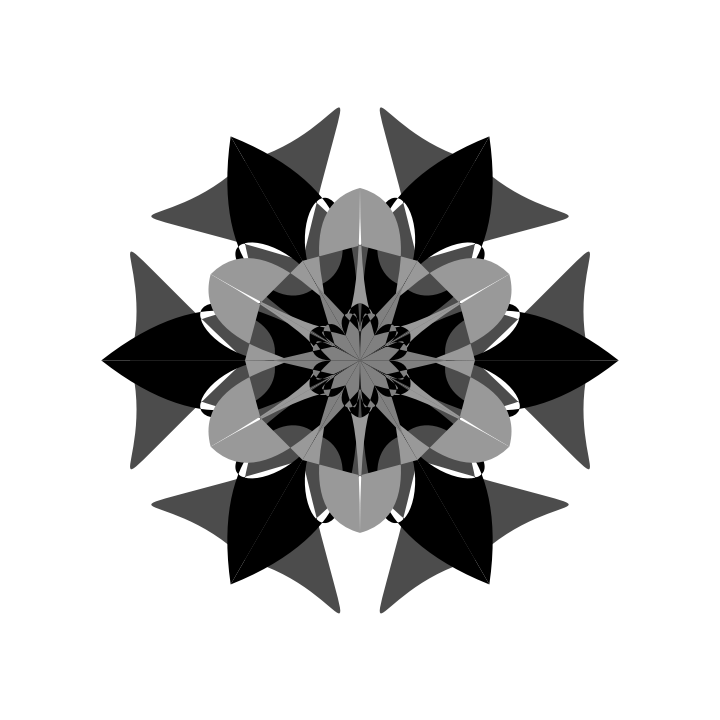Using the option ColorFunction, colorized mandalas are obtained:

 In:=Out=#### RotationalSymmetryOrder (2)

The "RotationalSymmetryOrder" option specifies how many copies of the seed segment comprise the mandala:

 In:=Out=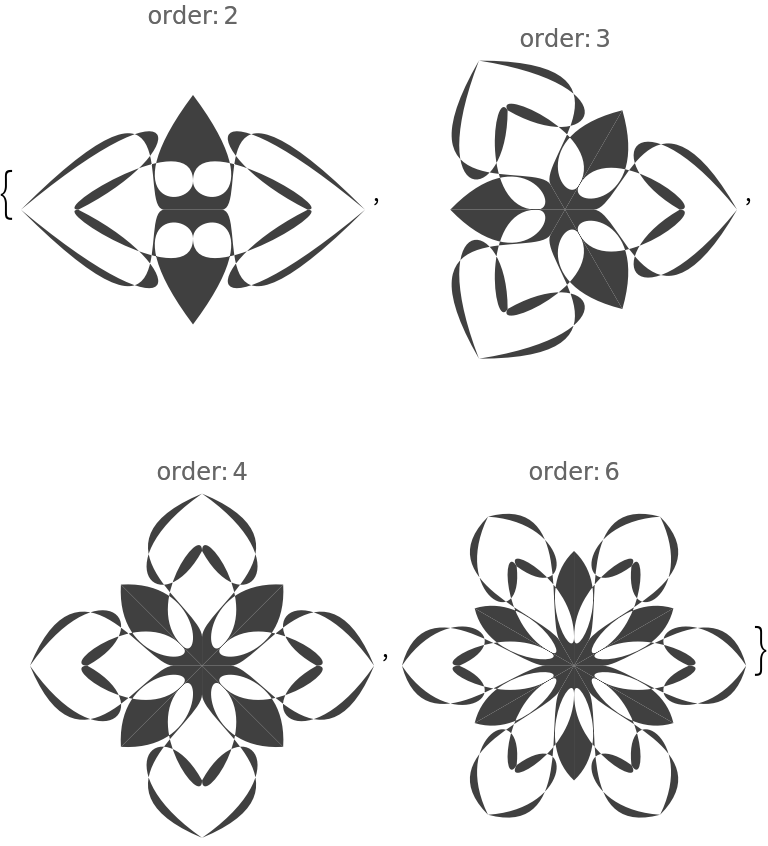In:=Out=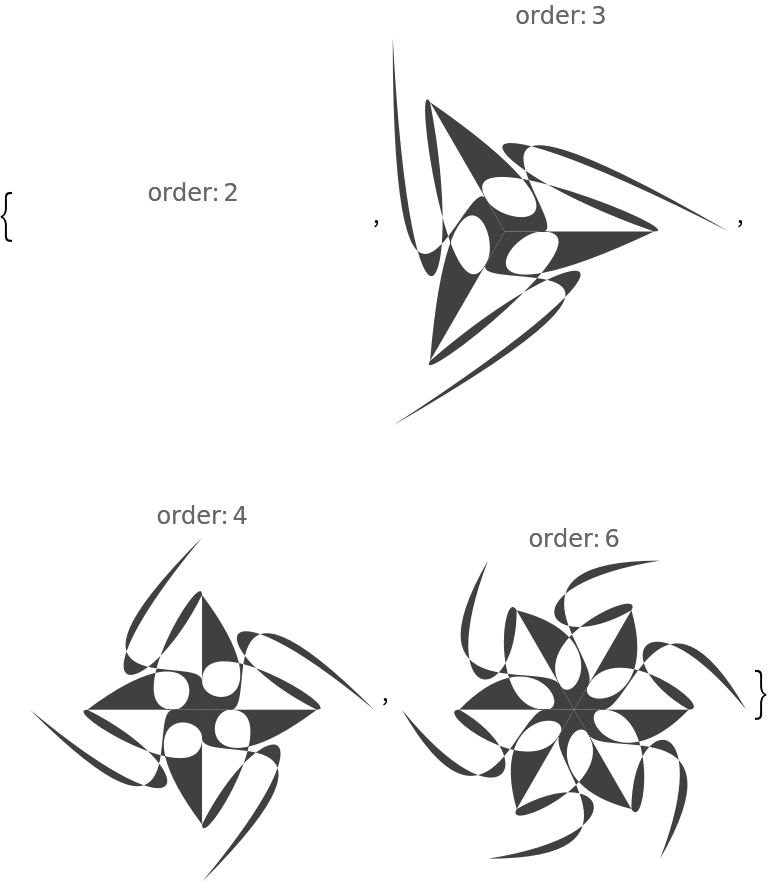If both "Radius" and "RotationalSymmetryOrder" are given lists of numbers, then the corresponding elements in those are paired to make the specifications of the colorized mandalas:

 In:=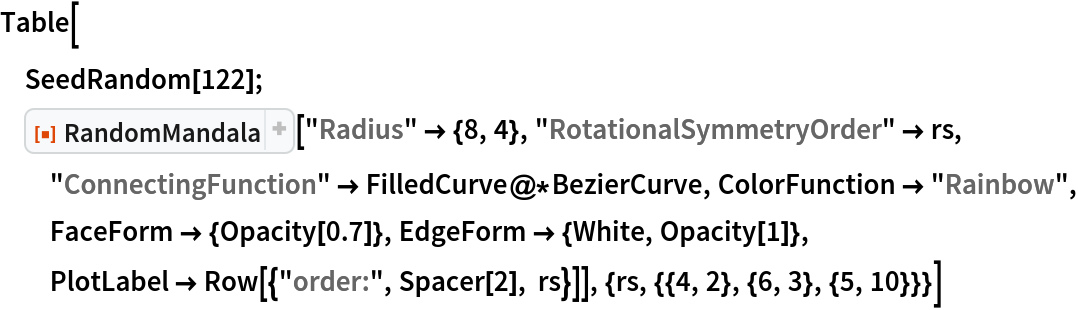Out=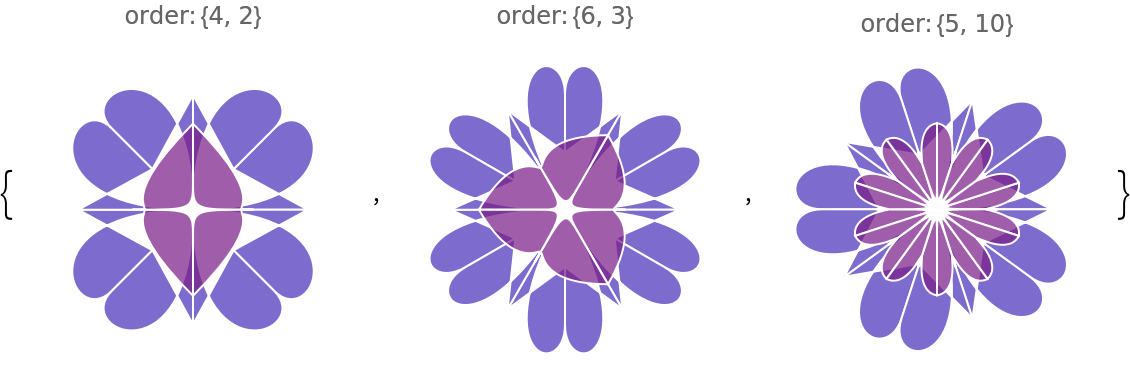#### SeedFunction (3)

The option "SeedFunction" specifies the function for drawing mandala's seed segment. If the value is Automatic, then the seed segment is drawn by taking into account the values of the options "Radius", "NumberOfSeedElements", "KeepGridPoints" and "ConnectingFunction".

Here is a custom seed function that places circles or disks at random points within the segment that corresponds to radius and angle:

 In:=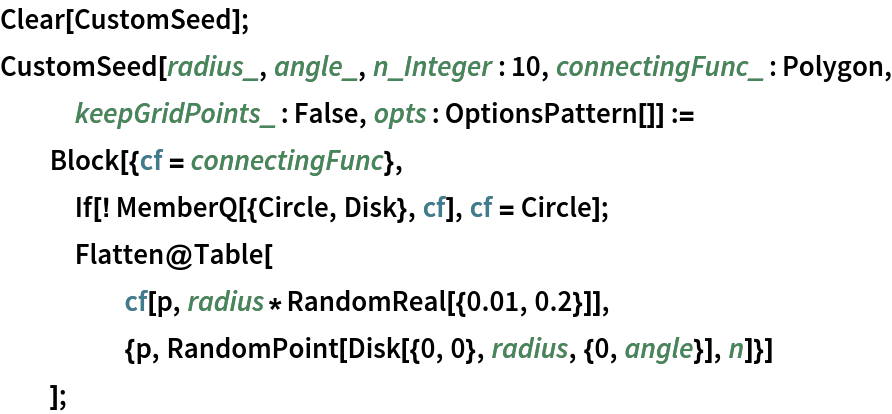Here are single-mandala mode mandalas made with the custom seed segment function defined above:

 In:=Out=Here are multi-mandala mode colorized mandalas (with two seed elements and randomly selected connecting functions):

 In:=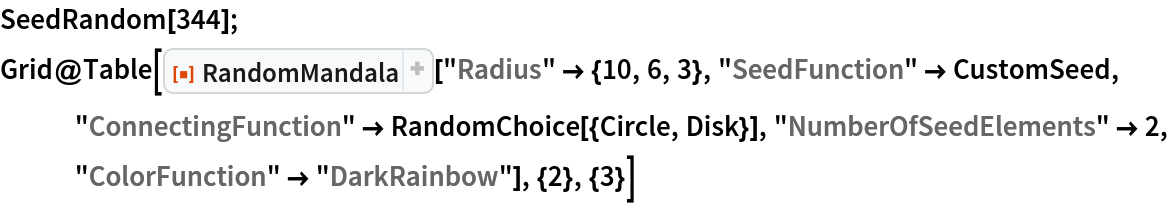Out=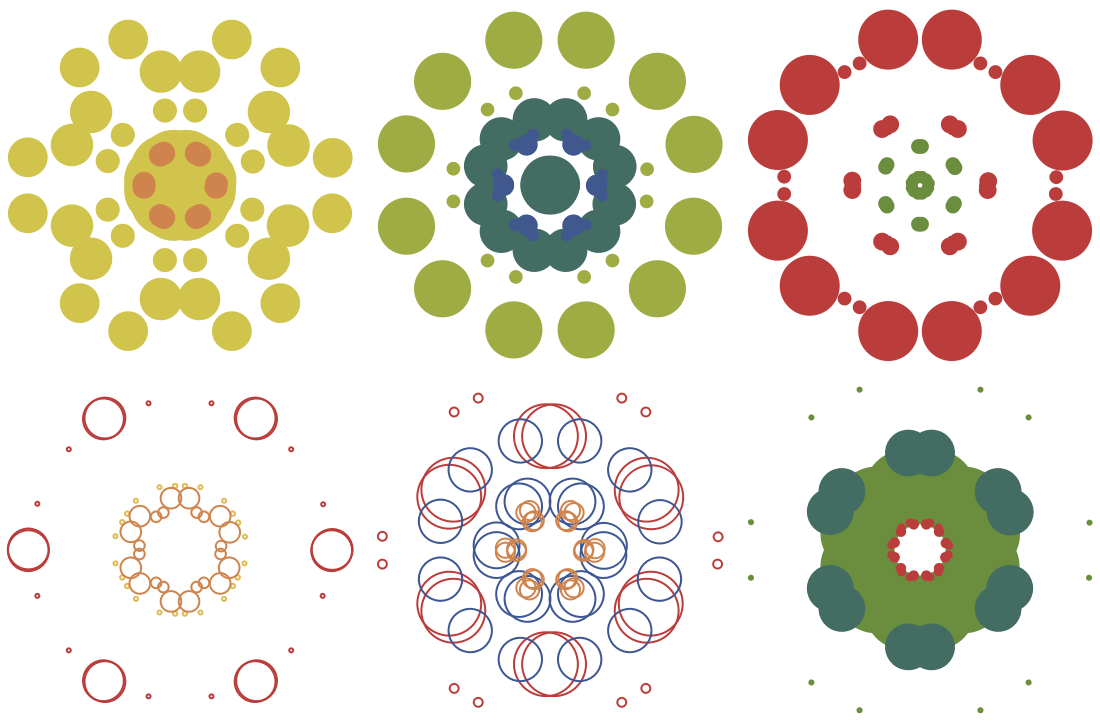#### SymmetricSeed (1)

The option "SymmetricSeed" specifies whether the seed segment should be symmetric:

 In:=Out=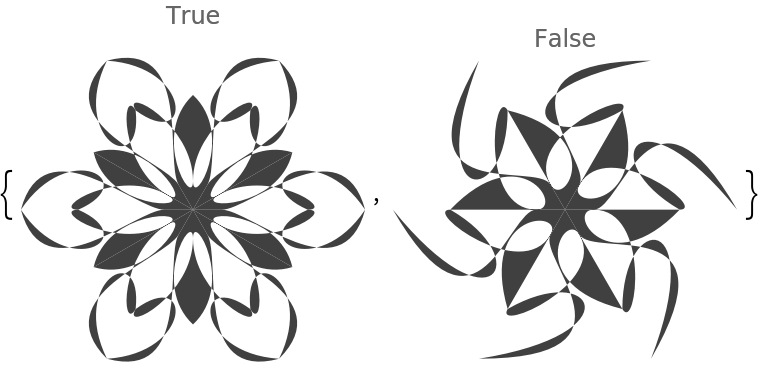### Properties and Relations (2)

Using combinations of settings for ColorFunction, EdgeForm and FaceForm can produce certain more nuanced mandala effects than, say, just using ColorFunction:

 In:=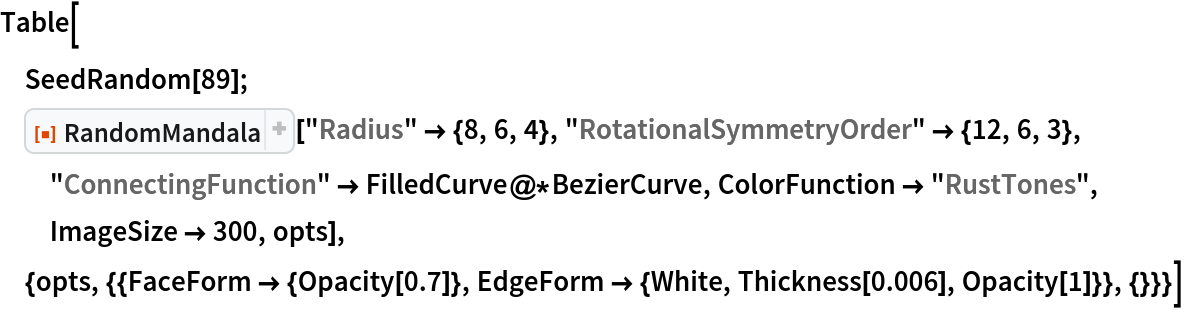Out=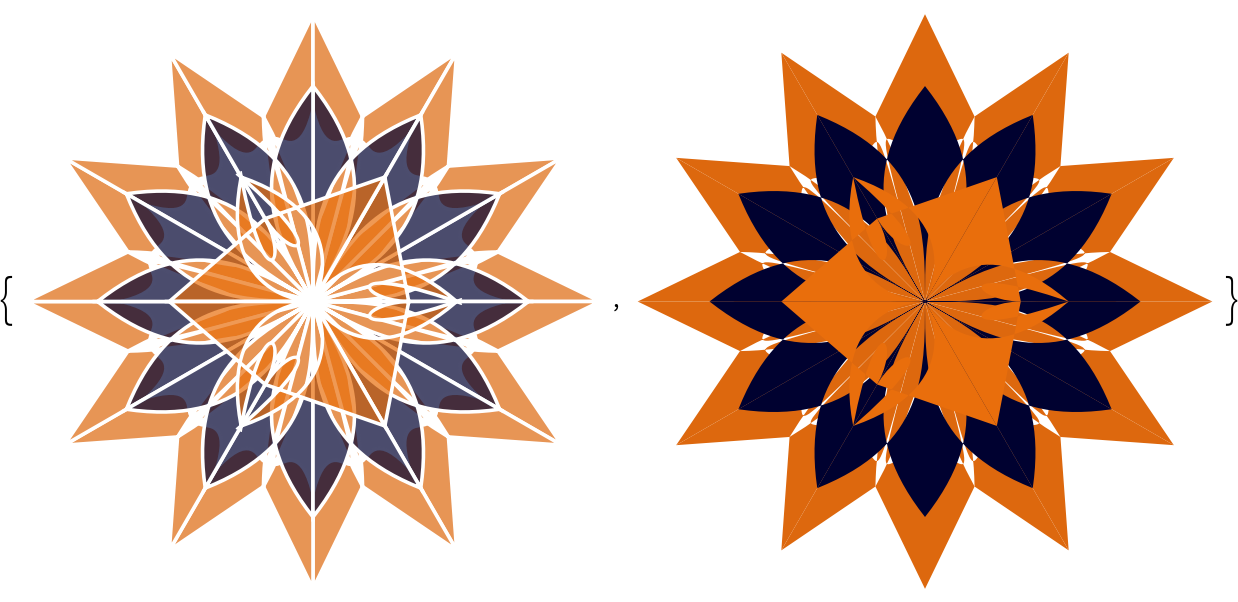RandomMandala can be used in more or less the same way as the resource function RandomScribble; here RandomMandala is used with the resource function TexturizePolygons:

 In:=Out=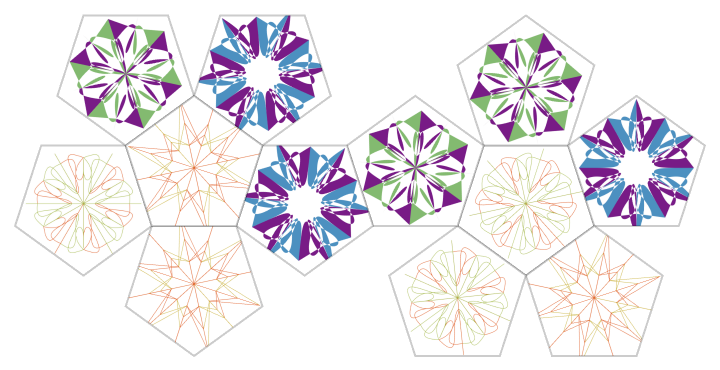Here the resource function RandomScribble is used with the resource function TexturizePolygons:

 In:=Out=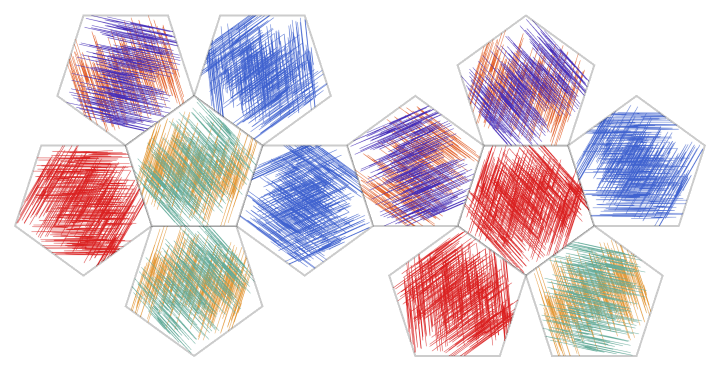### Possible Issues (1)

If the specified rotational symmetry order is not an integer, then the produced mandalas will appear "open":

 In:=Out=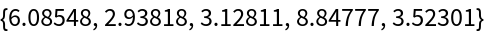In:=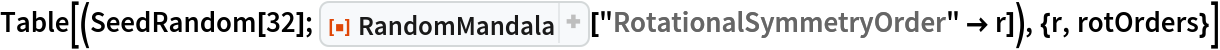Out=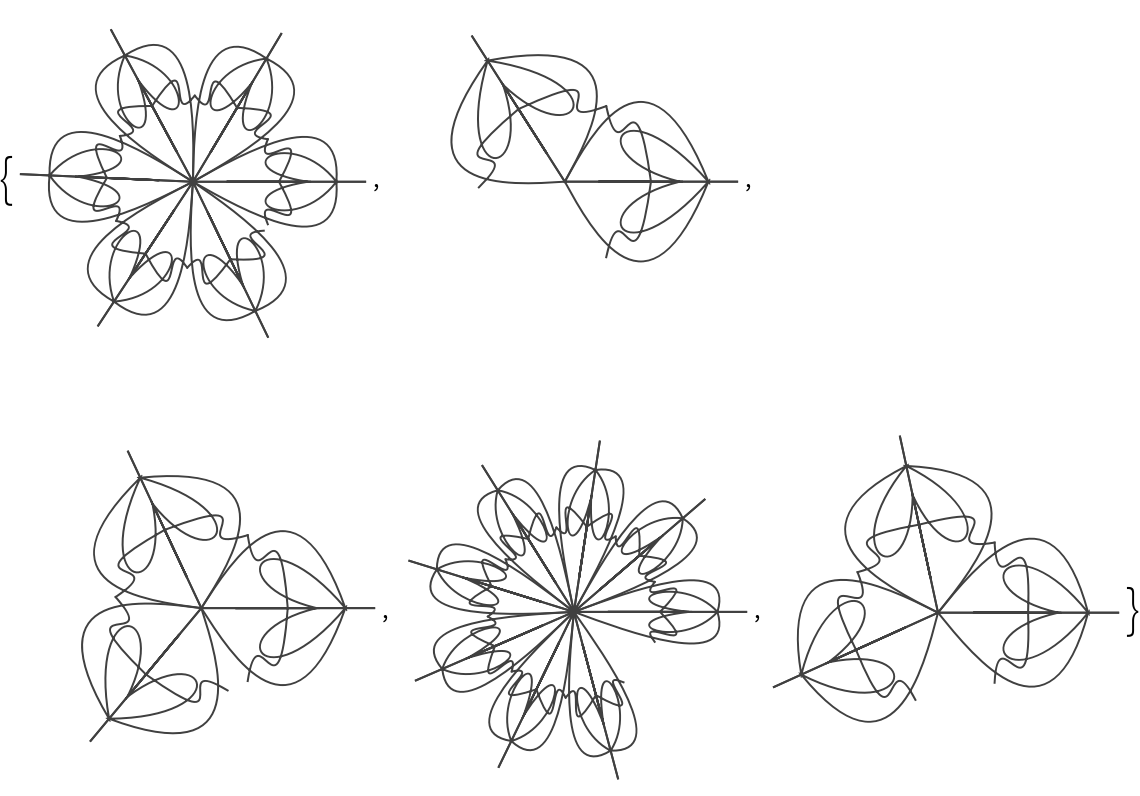In:=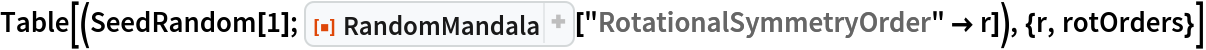Out=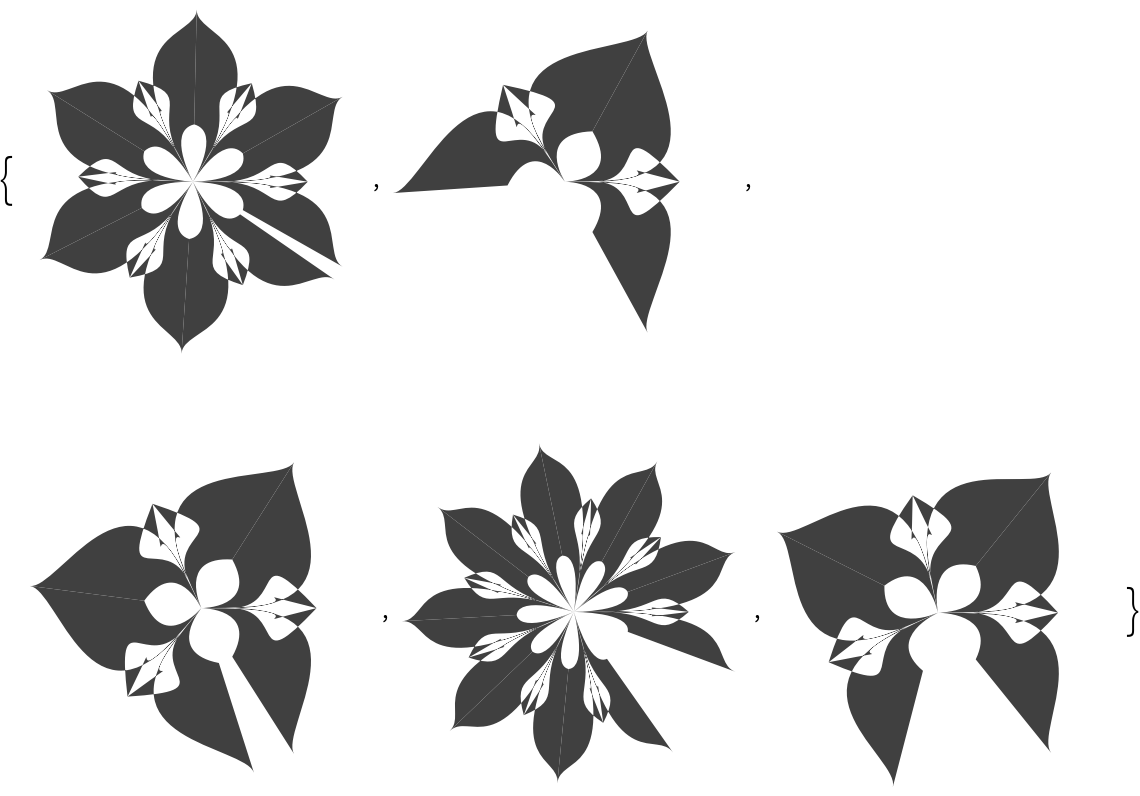### Neat Examples (8)

#### Random mandalas (3)

A table of random mandalas:

 In:=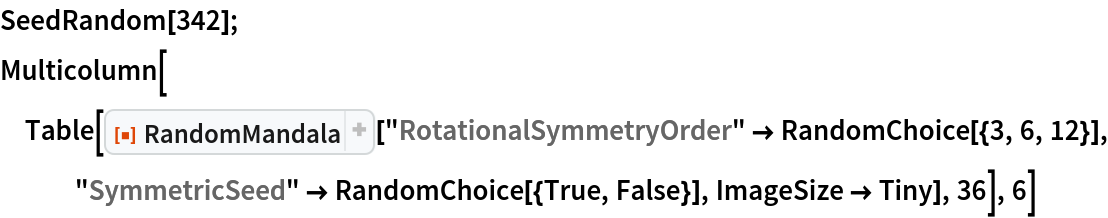Out=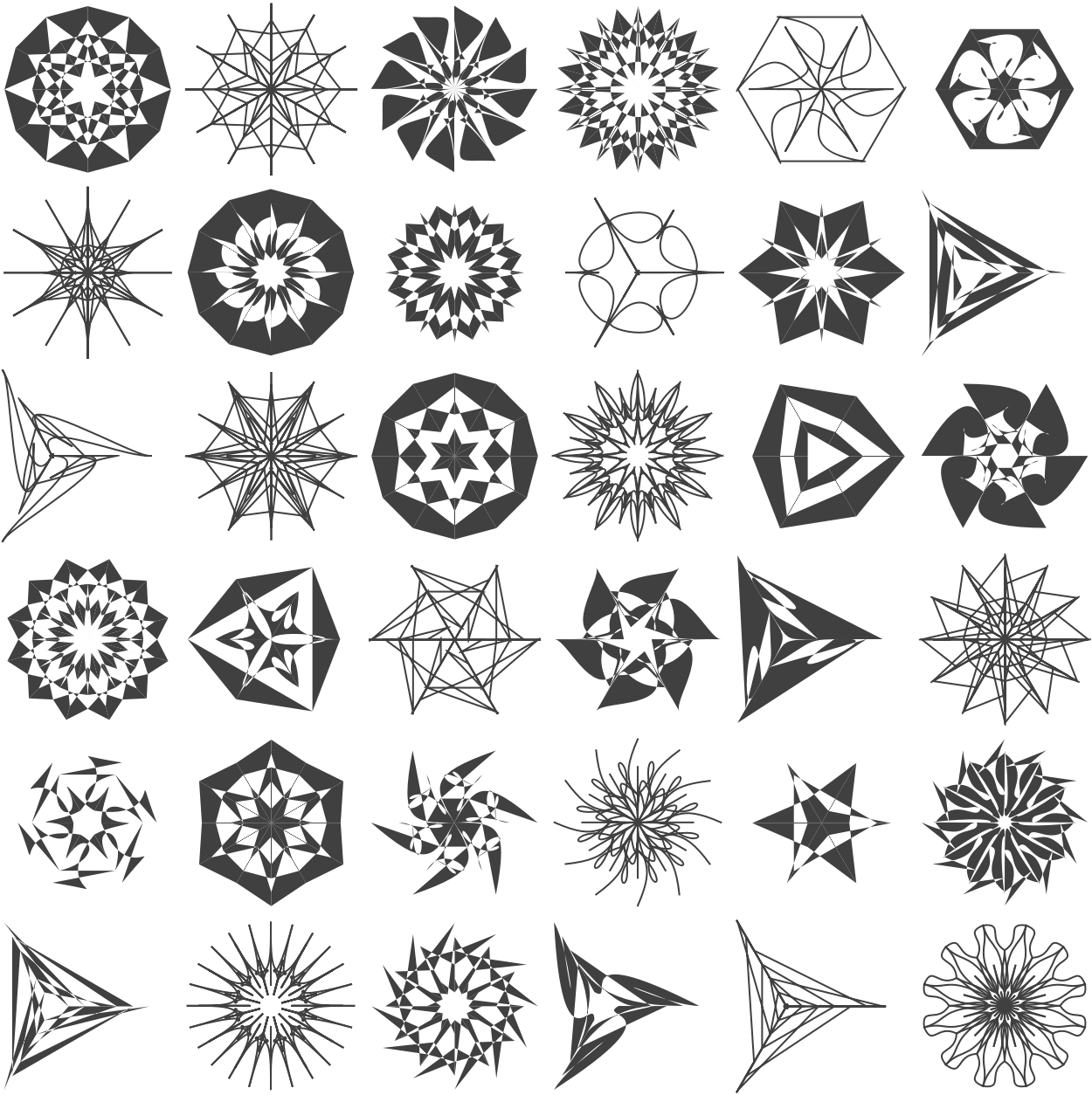A table of random colorized mandalas:

 In:=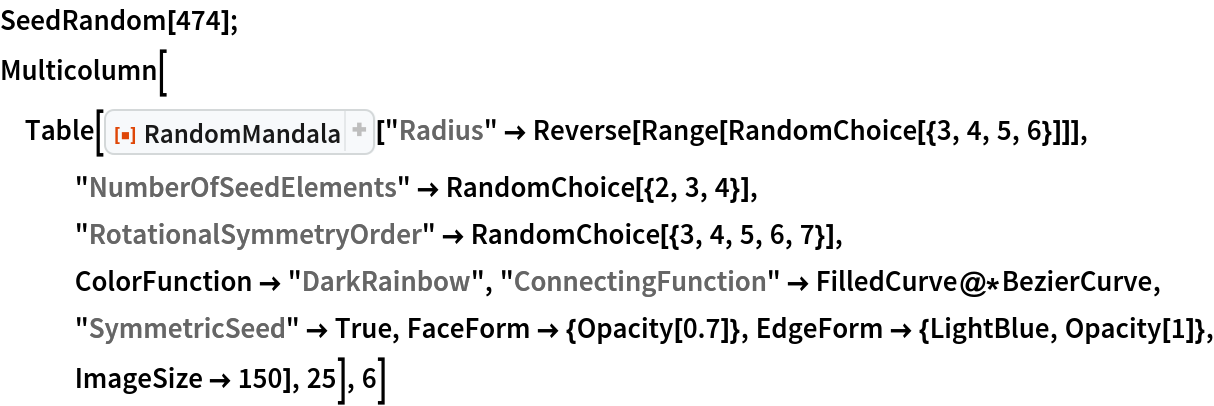Out=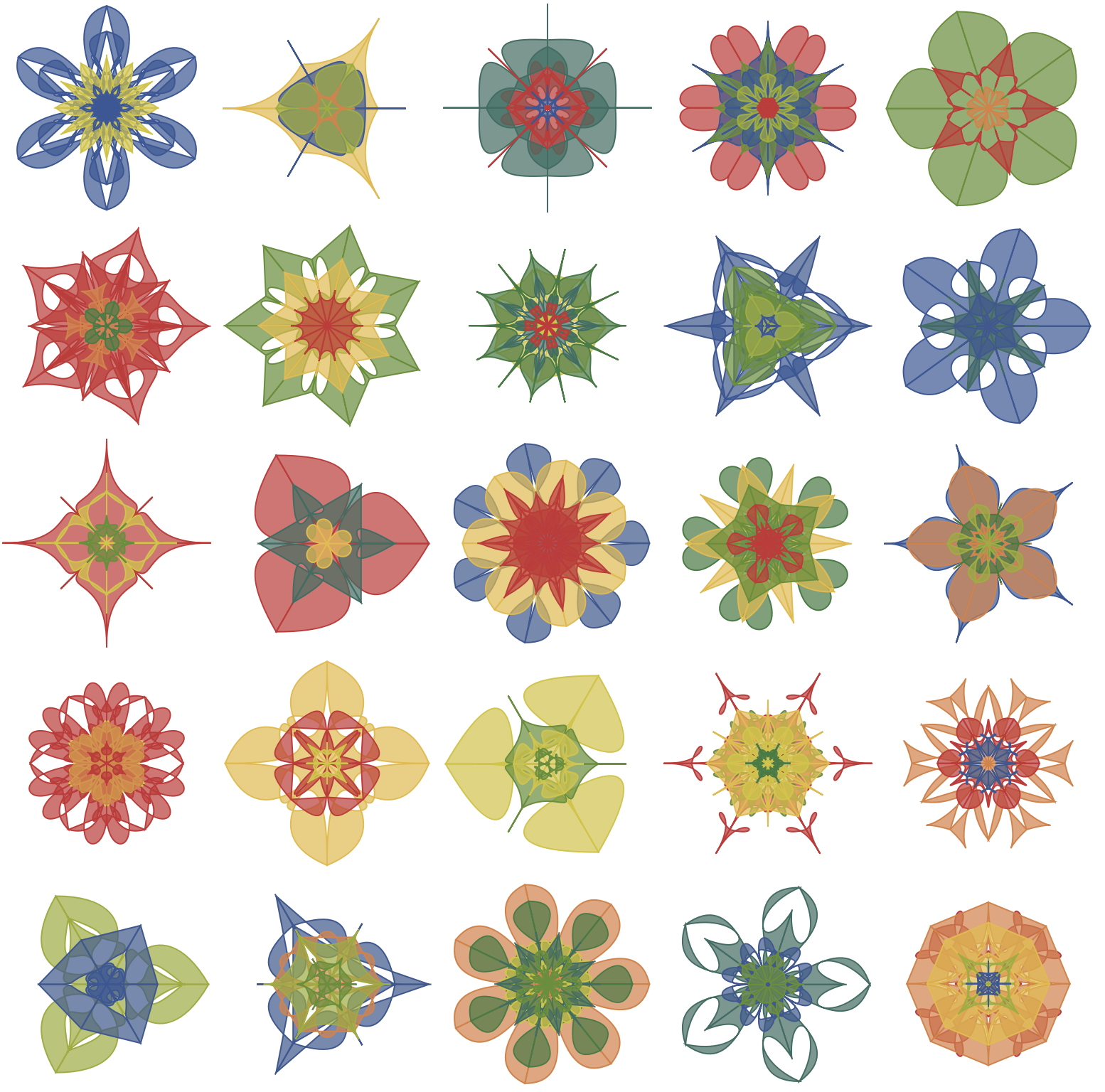A table of "open" colorized mandalas:

 In:=Out=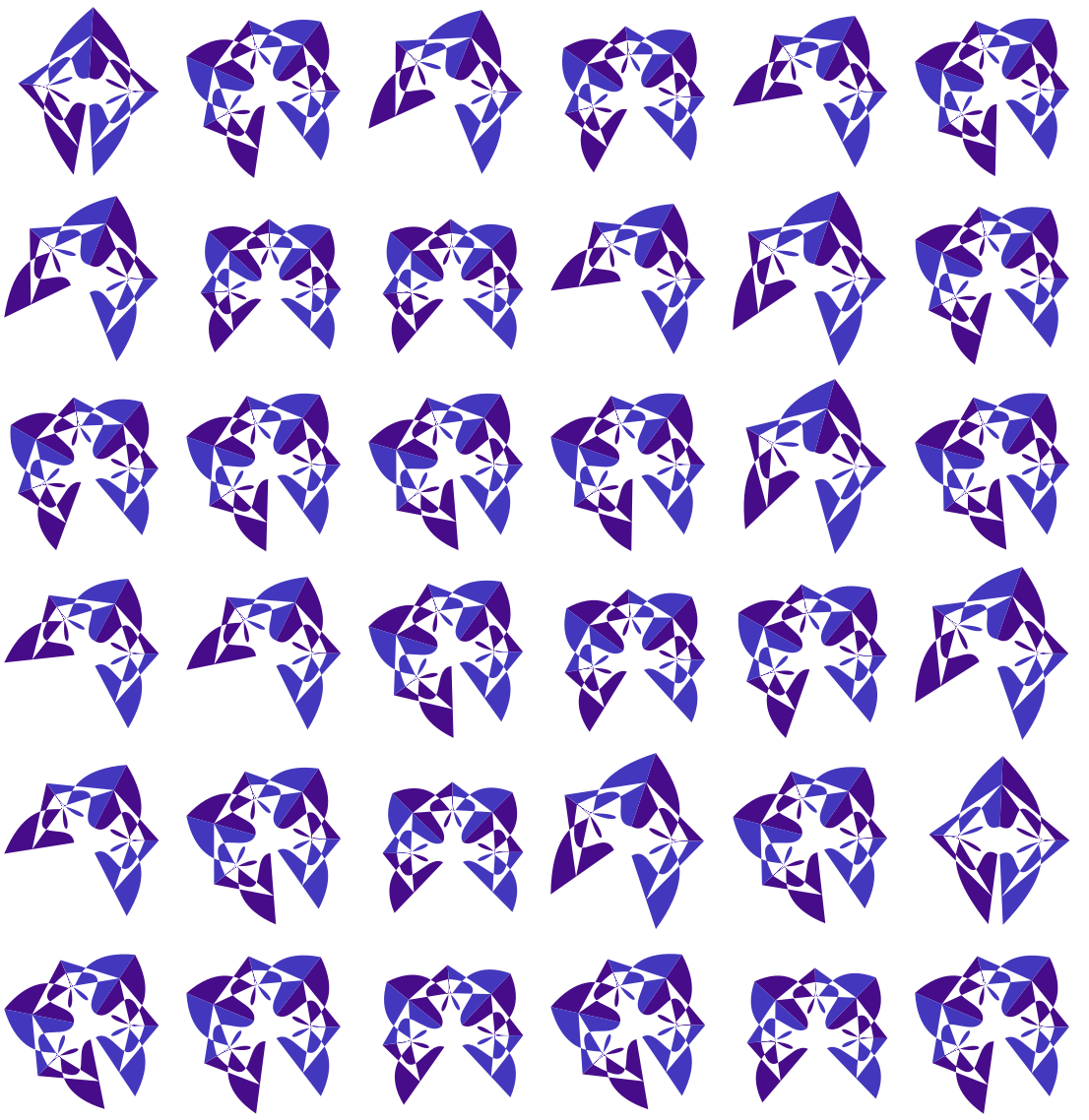#### Colored mandala images by blending (4)

Make a list of random mandalas:

 In:=Out=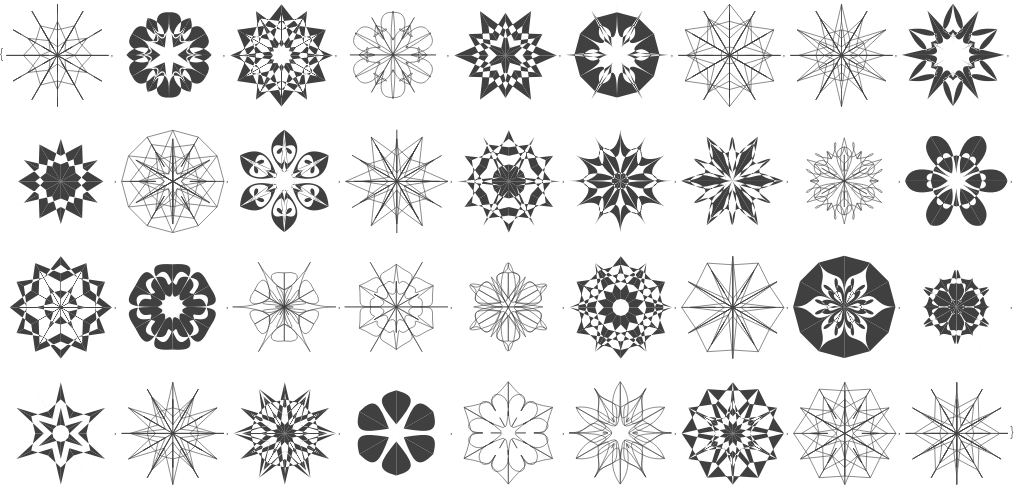Make images of the generated mandala graphics:

 In:=Out=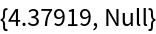Pick mandala images at random and blend them using a set of coloring schemes:

 In:=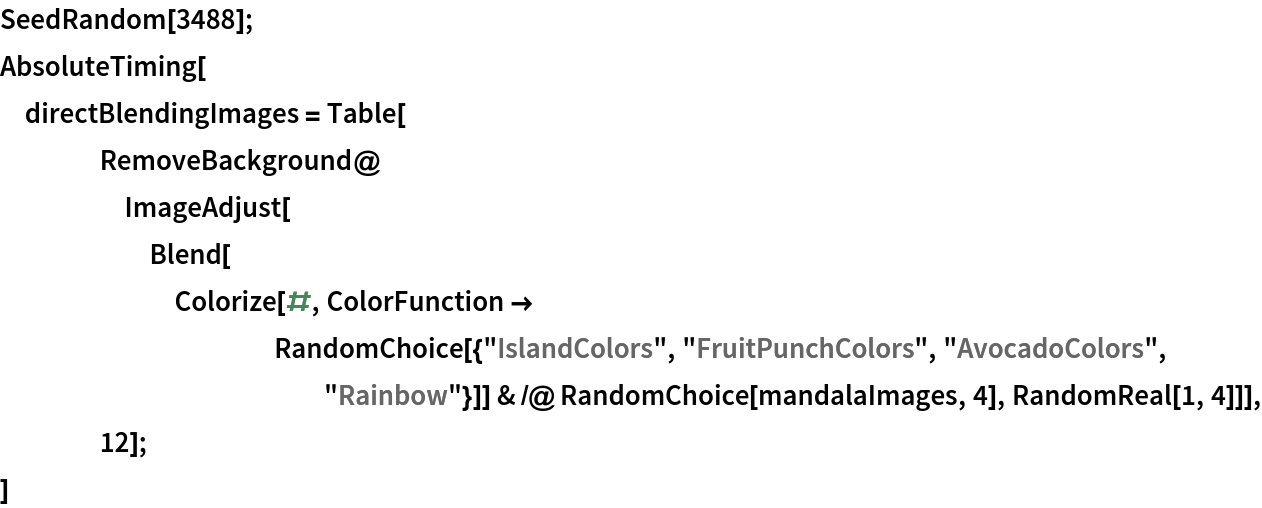Out=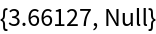Make a collage:

 In:=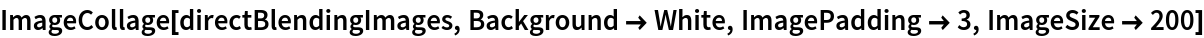Out=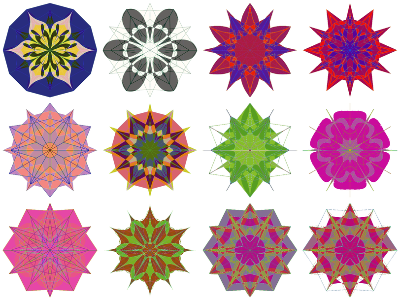#### Mandalas made with random scribbles (1)

Here are mandalas made with the repository function RandomScribble:

 In:=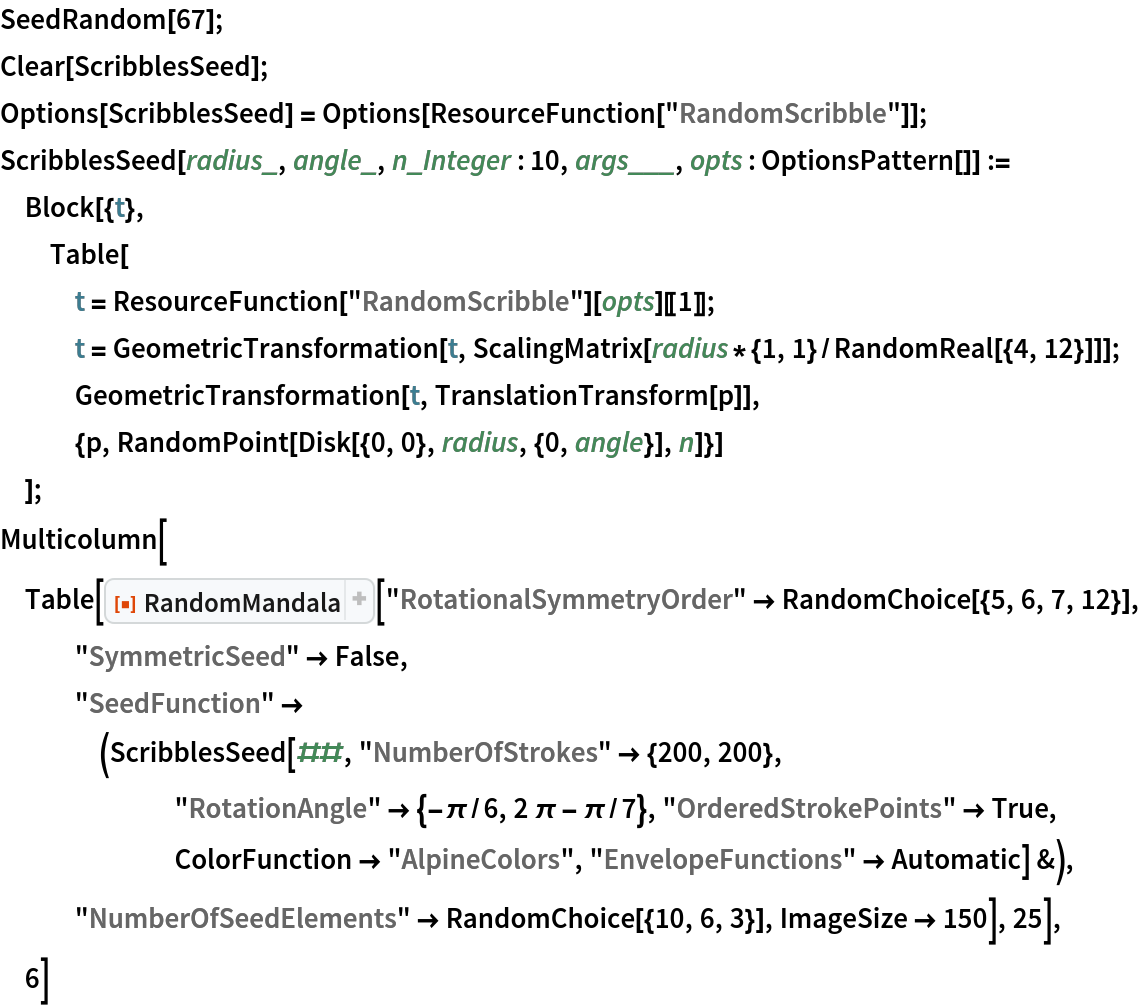Out=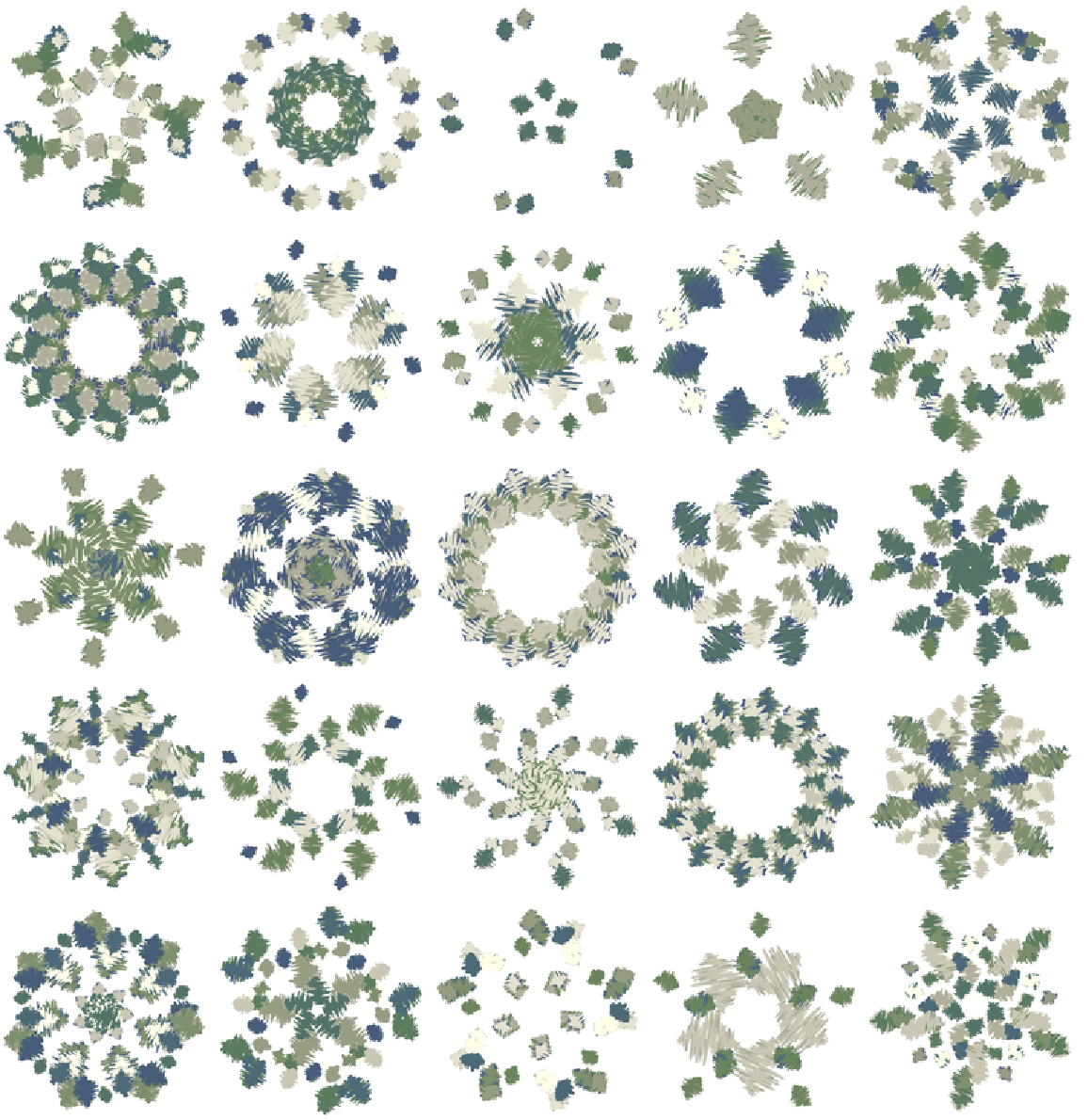## Author Notes

The Python RandomMandala package was implemented in order to compare it with this repository function (and, hence, compare Python and the Wolfram Language).